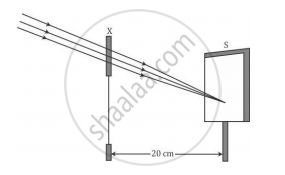Share

# A Student Focused the Sun Rays Using an Optical Device 'X' on a Screen S as Shown. - CBSE Class 10 - Science

ConceptReflection of Light Spherical Mirrors

#### Question

A student focused the Sun rays using an optical device 'X' on a screen S as shown.From this it may be concluded that the device 'X' is a (select the correct option)

(A) Convex lens of focal length 10 cm.

(B) Convex lens of radius of curvature 20 cm.

(C) Convex lens of focal length 20 cm.

(D) Concave mirror of focal length 20 cm.

#### Solution

Light rays from infinity are converged at the focal point of the convex lens. Therefore, the focal length of the convex lens is 20 cm.

Hence, the correct option is C.

Is there an error in this question or solution?

#### Video TutorialsVIEW ALL 

Solution A Student Focused the Sun Rays Using an Optical Device 'X' on a Screen S as Shown. Concept: Reflection of Light - Spherical Mirrors.
S